# PSEB 9th Class Maths Solutions Chapter 7 Triangles Ex 7.2

Punjab State Board PSEB 9th Class Maths Book Solutions Chapter 7 Triangles Ex 7.2 Textbook Exercise Questions and Answers.

## PSEB Solutions for Class 9 Maths Chapter 7 Triangles Ex 7.2

Question 1.
In an isosceles triangle ABC, with AB = AC, the bisectors of ∠ B and ∠ C intersect each other at O. Join A to O. Show that:
(i) OB = OC
(ii) AO bisects ∠ A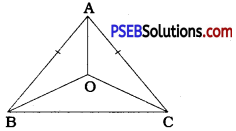In ∆ ABC, AB = AC
∴ ∠ ABC = ∠ ACB (Theorem 7.2)
∴ $$\frac{1}{2}$$ ∠ ABC = $$\frac{1}{2}$$ ∠ ACB
∴ ∠ OBC = ∠ OCB (BO bisects ∠ ABC and CO bisects ∠ ACB)
Now, in ∆ OBC, ∠ OBC = ∠ OCB
∴ OB = OC (Theorem 7.3)
Similarly, ∠ ABC = ∠ ACB gives
∴ $$\frac{1}{2}$$ ∠ ABC = $$\frac{1}{2}$$ ∠ ACB
∴ ∠ ABO = ∠ ACO
Now, in ∆ ABO and ∆ ACO,
AB = AC (Given)
∠ ABO = ∠ ACO
and OB = OC
∴ ∆ ABO ≅ ∆ ACO (SAS rule)
∴ ∠ BAO = ∠ CAO (CPCT)
But, ∠ BAO + ∠ CAO = ∠ BAC (Adjacent angles)
∴ ∠ BAO = ∠ CAO = $$\frac{1}{2}$$ ∠ BAC
Thus, AO bisects ∠ A.Question 2.
In ∆ ABC, AD is the perpendicular bisector of BC (see the given figure). Show that ∆ ABC is an isosceles triangle in which AB = AC.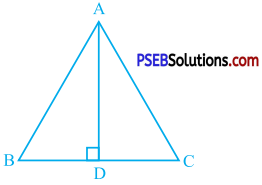In ∆ ABC, AD is the perpendicular bisector of BC.
∴ BD = CD and ∠ ADB = ∠ ADC = 90°
and BD = CD
∴ AB = AC (CPCT)
Now, in ∆ ABC, AB = AC.
Hence, ∆ ABC is an isosceles triangle in which AB = AC.Question 3.
ABC is an isosceles triangle in which altitudes BE and CF are drawn to equal sides AC and AB respectively (see the given figure). Show that these altitudes are equal.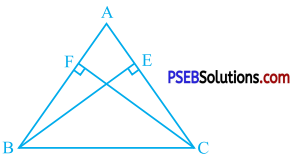In ∆ ABC, AC = AB
∴ ∠ ABC = ∠ ACB
∴ ∠ FBC = ∠ ECB
Now, in ∆ FBC and ∆ ECB,
∠ FBC = ∠ ECB
∠ BFC = ∠ CEB (Right angles)
BC = CB (Common)
∴ ∆ FBC ≅ ∆ ECB (AAS rule)
∴ CF = BE (CPCT)
Thus, the altitudes CF and BE on equal sides AB and AC respectively of ∆ ABC are equal.Question 4.
ABC is a triangle in which altitudes BE and CF to sides AC and AB are equal (see the given figure). Show that
(i) ∆ ABE ≅ ∆ ACF
(ii) AB = AC, i.e., ABC is an isosceles triangle.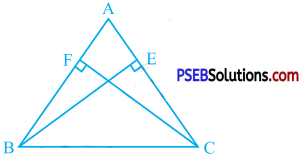In ∆ ABE and ∆ ACF,
∠ AEB = ∠ AFC (Right angles)
∠ A = ∠ A (Common)
BE = CF (Given)
∴ ∆ ABE ≅ ∆ ACF (AAS rule)
∴ AB = AC (CPCT)
Thus, ∆ ABC is an isosceles triangle.

Question 5.
ABC and DBC are two isosceles triangles on the same base BC (see the given figure). Show that ∠ ABD = ∠ ACD.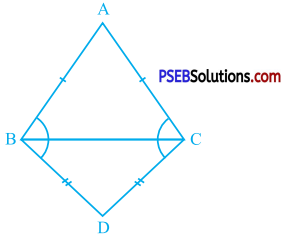∠ ABC and ∠ DBC are adjacent angles.
∴ ∠ ABC + ∠ DBC = ∠ ABD ………… (1)
∠ ACB and ∠ DCB are adjacent angles.
∴ ∠ ACB + ∠ DCB = ∠ ACD ………….. (2)
In ∆ ABC, AB = AC.
∴ ∠ ABC = ∠ ACB (Theorem 7.2)
In ∆ DBC, DB = DC.
∴ ∠ DBC = ∠ DCB (Theorem 7.2)
∴ ∠ ABC + ∠ DBC = ∠ ACB + ∠ DCB
∴ ∠ ABD = ∠ ACD [From (1) and (2))Question 6.
∆ ABC is an isosceles triangle in which AB = AC. side BA is produced to D such that AD = AB (see the given figure). Show that ∠ BCD is a right angle.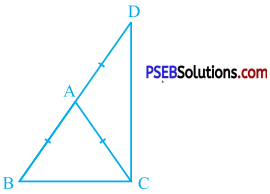AB = AC and AD = AB
In ∆ ABC, AB = AC
∴ ∠ ACB = ∠ ABC (Theorem 7.2) ……………… (1)
∴ ∠ ACD = ∠ ADC (Theorem 7.2) ……………… (2)
∠ ACB + ∠ ACD = ∠ ABC + ∠ ADC
∴ ∠ BCD = ∠ DBC + ∠ BDC
(Adjacent angles and A lies on BD)
In ∆ BCD,
∠ DBC + ∠ BDC + ∠ BCD = 180°
∴ ∠ BCD + ∠ BCD = 180° (from (3)]
∴ 2 ∠ BCD = 180°
∴ ∠ BCD = 90°
Thus, ∠ BCD is a right angle.Question 7.
ABC is a right angled triangle in which ∠A = 90° and AB = AC. Find ∠ B and ∠ C.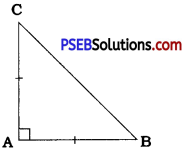In ∆ ABC, AB = AC
∴ Z C = Z B (Theorem 7.2)
In ∆ ABC,
∠ A + ∠ B + ∠ C = 180°
∴ 90° + ∠ B + ∠ B = 180° (Given and ∠ C = ∠ B)
∴ 2 ∠ B = 90°
∴ ∠ B = 45°
∴ ∠ C = 45°Question 8.
Show that the angles of an equilateral triangle are 60° each.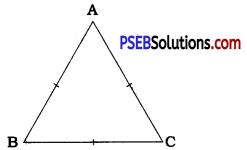∴ ∠ A = ∠ B = ∠ C = $$\frac{180^{\circ}}{3}$$ = 60°